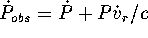Next: Binary Neutron Stars Up: A Tutorial on Radio Previous: Timing noise

# Assignment 4

1. If a timing model for a pulsar is complete except for the period derivative, what is the functional form of the residuals that you would expect? If a timing model for a pulsar is complete except for the period second derivative, what is the functional form of the residuals that you would expect?
2. Calculate the amplitude of the Shapairo delay in a double neutron star system that has an orbital inclination of 88 degrees.
3. Derive an expression for the proper motion contribution to the period derivative of a pulsar in terms of the transverse velocityand distance d. Recallwhereis the radial velocity.
4. Estimate how many times the Crab pulsar has rotated since its discovery. Estimate how many times PSR B1937+21 has rotated since its discovery.
5. To what accuracy do we need to know the position of the Lovell telescope in order to measure pulse arrival times to an accuracy of 1 microsecond?
6. What is the probability of catching a glitch occuring in the Crab pulsar. ie for what fraction of a day is the Crab pulsar observable from Jodrell bank.

Jon Bell
Thu Dec 19 15:15:11 GMT 1996